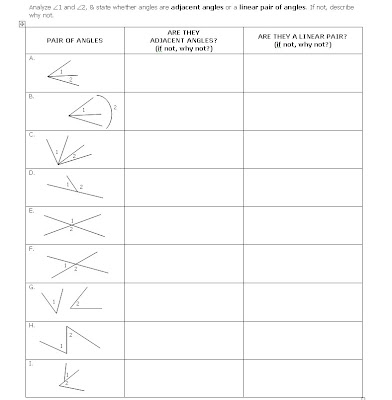## Wednesday, October 20, 2010

### Angle Pairs

We're starting geometric proofs now, and yesterday we did two activities to prep for this. First, I wanted the students to be familiar with some basic definitions and to know that they are biconditionals, and see how they're used as reasons for proofs. Second, I wanted the students to look at partial pieces of proofs and fill in the blanks with reasons, or steps if given the reasons.

Here's an activity I did to get them thinking about adjacent angles, linear pairs of angles, and vertical angles:We first looked at the definition of adjacent angles. Then I had them go down that column and either indicate YES, or if NO, then list the parts of the definition it violated. Then we did the same with linear pair. Then I just had them write on the LHS which one(s) were vertical angles.

Next we took notes on how theorems, definitions, postulates, properties could be used as proof reasons, and I had them refer to a print out of our basic ones so far (along with definitions), and do the following:The pesky bell rang, and we ran out of time, so I'll have to see how they did next class.

1.http://TrianCal.esy.es - Open in Google Chrome. (Triangles online calculator developed by Jesus S.)

I propose this free online calculator triangles without advertising to help students with geometry, does not perform the duties, because their calculations formulas are not displayed. It is designed in a didactic way to check and view the realized duties.

TrianCal is online calculator triangles that works with any combination of values including sides, heights, angles, the area or perimeter of any triangle, calculating it with the minimum possible value (typically three).

Other functions:
- Draw the triangle (s) with GeoGebra.
- Set the range of values in each element.
- The type of angle.
- The type of triangle by its angles and sides.
- Selection of language (English or Spanish).
- Select the angle type [degrees (°), radians, degrees, minutes and seconds (° ' ") or degrees and minutes (° ')].
- Number of decimal places shown in the results (0-15).
- You can use the arrow keys and the Tab key to navigate through the settings.
- Drop-down menu to select the values comfortably.
- Create a link (URL) to the current triangle.
- An icon mail to communicate with the author.

NOTE: You must use the Google Chrome browser to display correctly TrianCal.

Examples of possible combinations:
- The area, perimeter and other data (side, height or angle), if the outside equilateral triangle would not need the third data.
- 2 angles and other data (if the value of the other data is not put aside the value of "a" at the time of drawing the triangle is 10).
- One side, one high and one angle.
- 3 heights.
- 3 sides.
- 2 heights and perimeter.
- Any other combination of values.

1.2.Choosing a room is like when we study. You should choose what you like or what you are good at. Otherwise, just like we play torture, we do n’t like it, not now, we will lose money for free. Useless
3.